Register for Maths, Science, English, Reasoning Olympiad Exams Register here | Book Free Demo for Live Olympiad Classes here | Check Olympiad Exam Dates here | Buy Practice Papers for IMO, IOM, HEO, IOEL etc here | Login here to participate in all India free mock test on every Saturday

# Length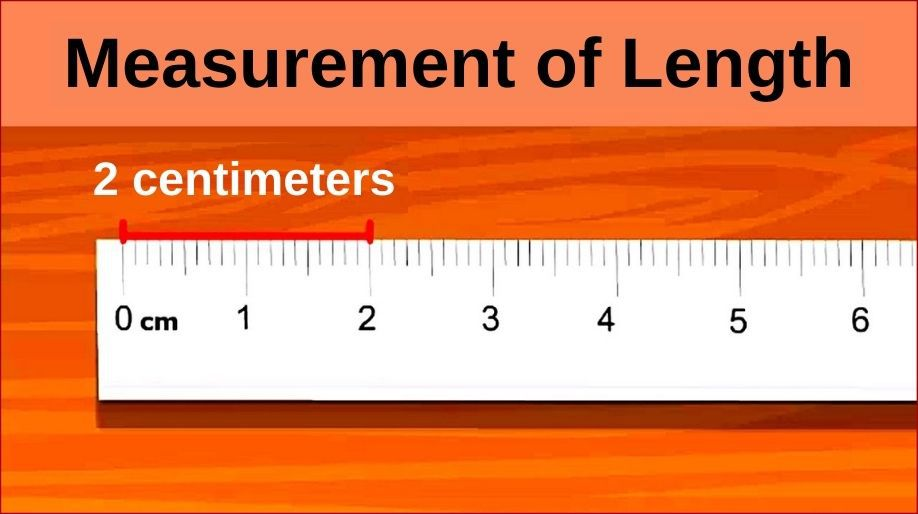What is length? Length is a measuring term which tells how long or wide an object is. Have you seen your pencil? Have you ever thought to measure how long it is? The answer to your question is Length. Let us know all about length in details.

Measurement of Length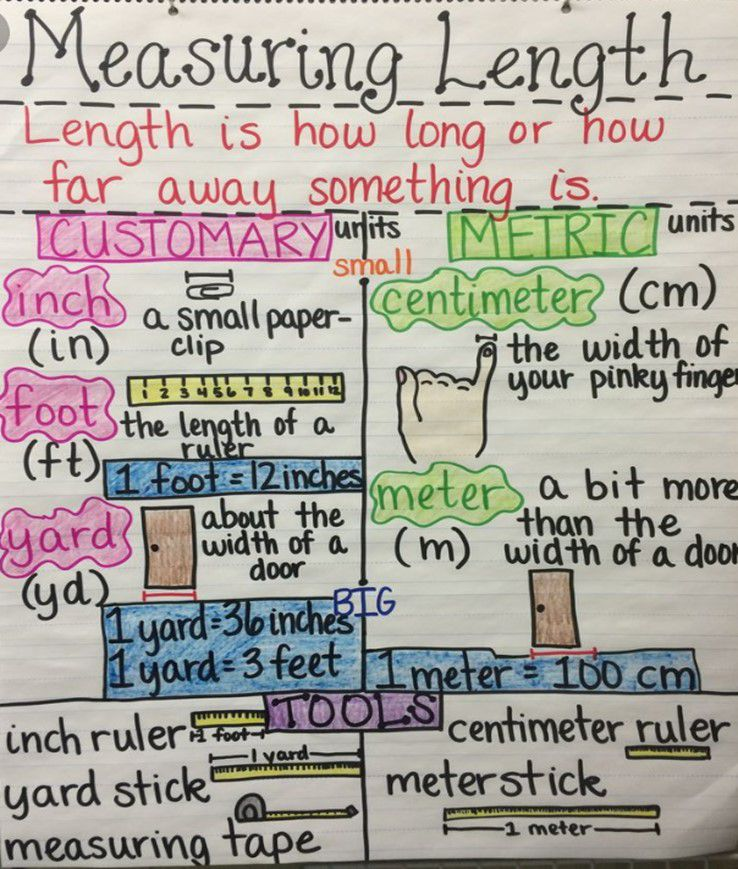Tools to Measure Length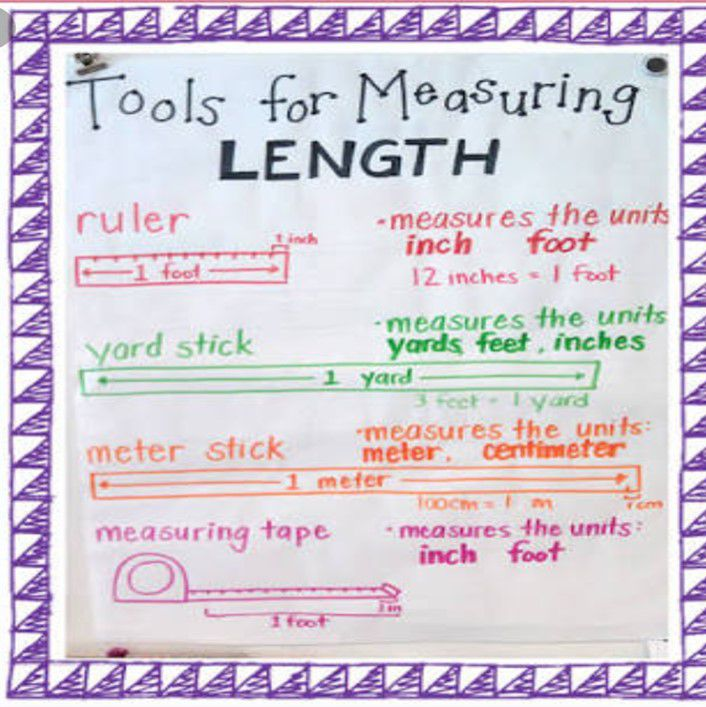Units to denote Length of body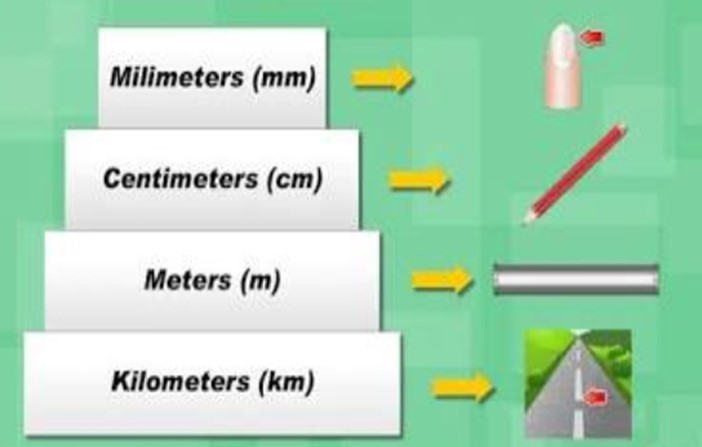Long or Tall Body

A body which has more length or more height is called longer or taller body. Example – Tree, Aeroplane, Train, etc.

Short Body

A body which is small in length and narrow in size is called a short body.

Example – Hen, pencil, pen, eraser, lizard, etc.

Practice Questions on Longer and Shorter Body

Solved examples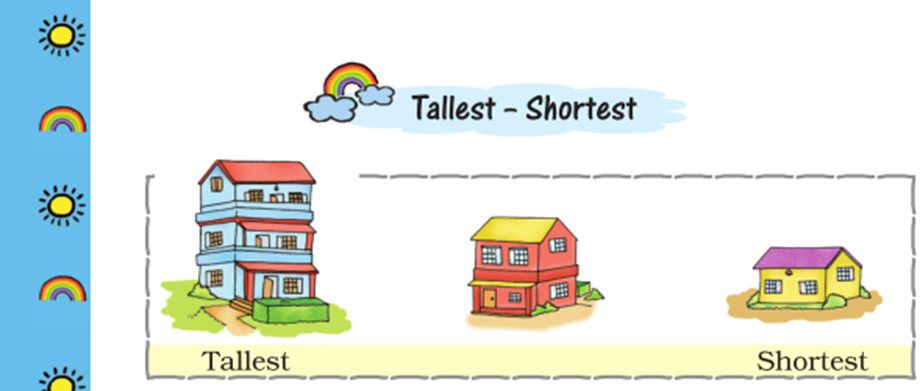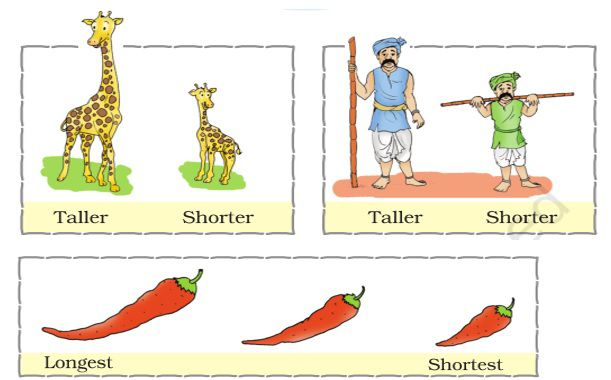Practice Questions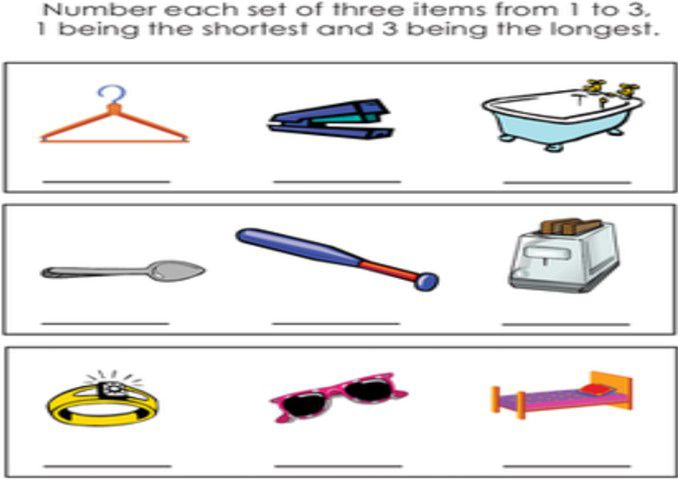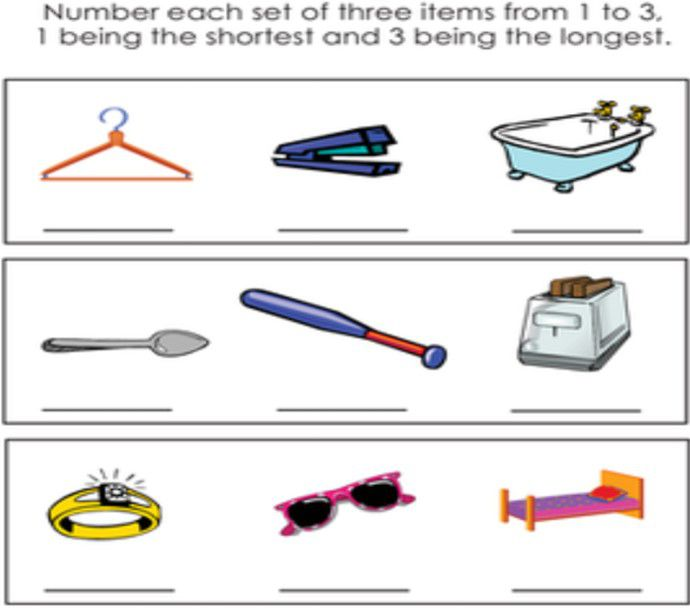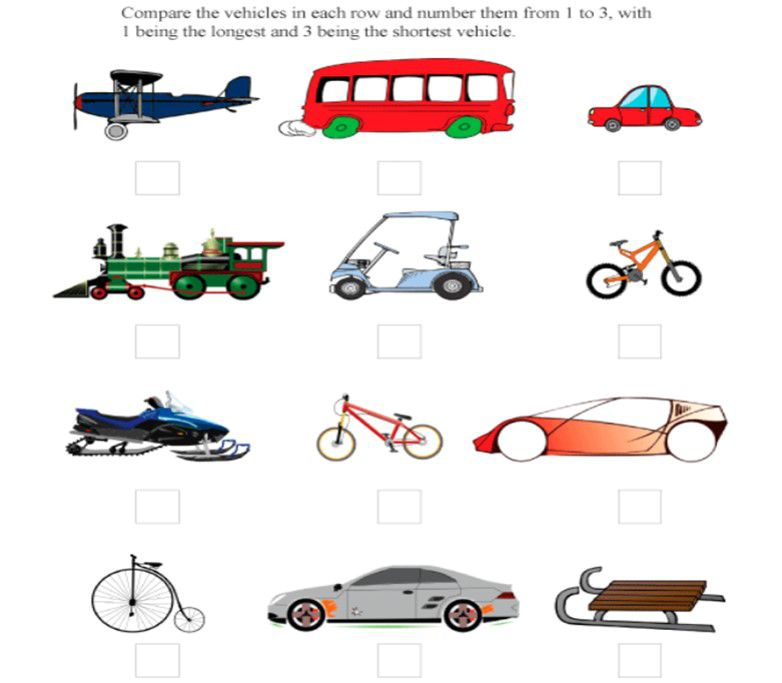Mark 1 to smallest body, 2 to longer body and 3 to longest body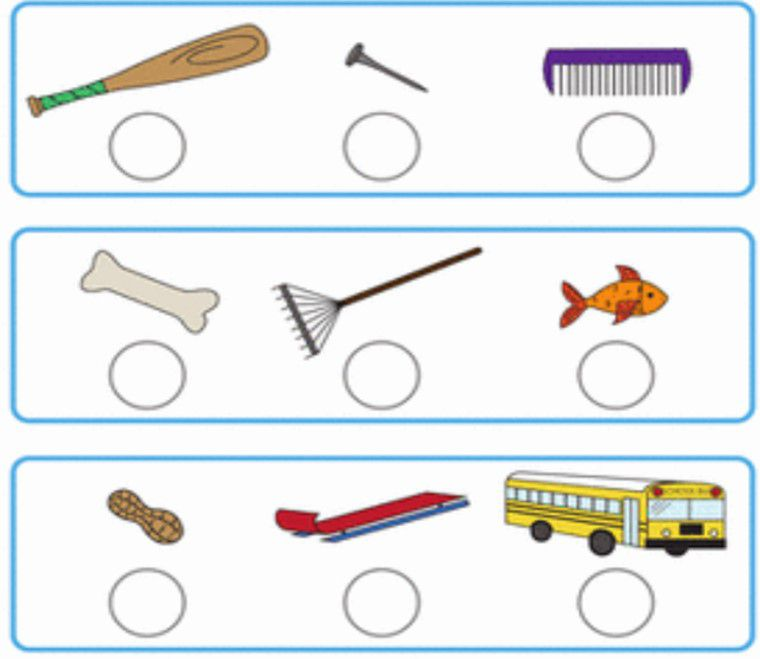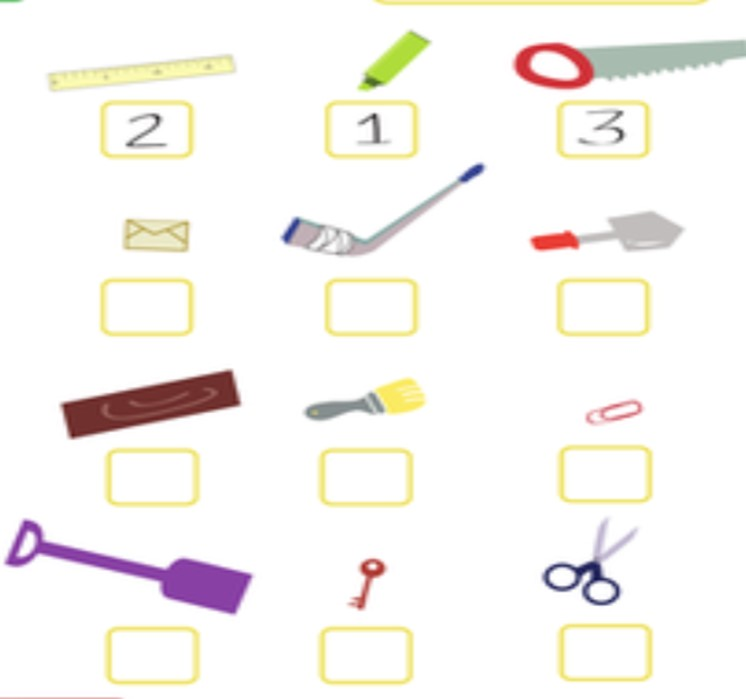Recap

• Length is the measuring term that tells how long or short an object is.
• Longer or taller body has more length or height. Example – Camel, Elephant, Train, etc.
• Shorter bodies are of the short length and narrow in size. Example – Ant, Pencil, Lizard, etc.
• A longer body has always more length than a shorter body and it is

big in size as compared to the small body.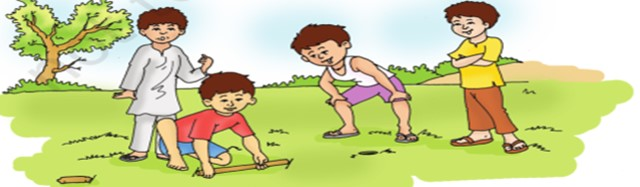## Quiz for Length

 Q.1 Look at the following image and answer the following questions: Which chicken is third nearest to the hen?a) W b) X c) Y d) Z
 Q.2 Look at the following image and answer the following questions: Which tree is taller than Tree R but shorter than Tree P?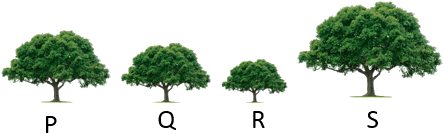a) P b) Q c) R d) S
 Q.3 Look at the following image and answer the following questions: Which spider is farthest away from wall?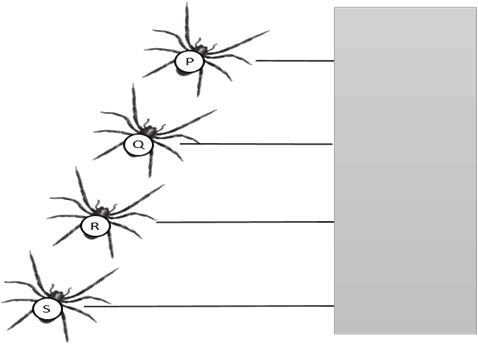a) P b) Q c) R d) S
 Q.4 Look at the following image and answer the following questions: Tape is ________ span long.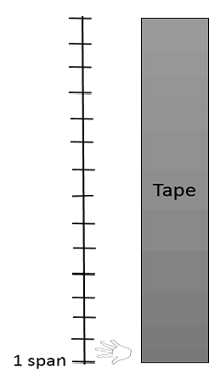a) 16 b) 15 c) 14 d) 13
 Q.5 Look at the following image and answer the following questions: Which is the shortest flower?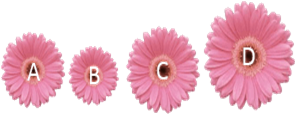a) A b) B c) C d) D
 Q.6 Look at the following image and answer the following questions: Which Penguin is nearest to the Snowman?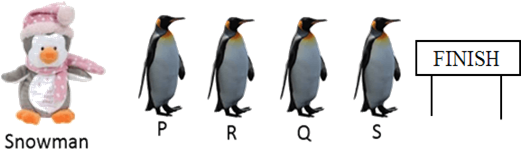a) P b) Q c) R d) S
 Q.7 Look at the following image and answer the following questions: Which bone is nearest to the dog?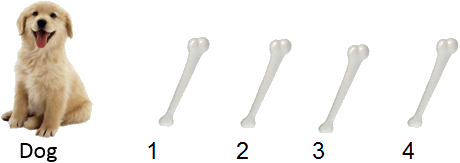a) 1 b) 2 c) 3 d) 4
 Q.8 Look at the following image and answer the following questions: Distance between two boys is ________ feet.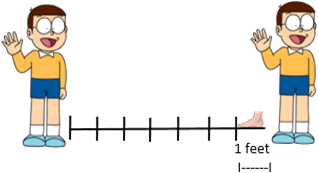a) 5 b) 10 c) 7 d) 6
 Q.9 Look at the following image and answer the following questions: Which of the following pigeon has the maximum height?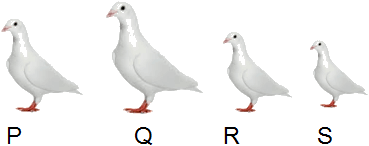a) P b) Q c) R d) S
 Q.10 Look at the following image and answer the following questions: If 1--------------> means 1 unit, then train is __________ units long.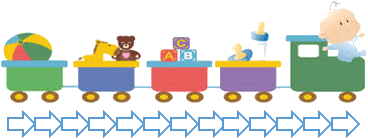a) 13 b) 18 c) 17 d) 12